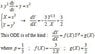# Tough differential equation

• suryanarayan

#### suryanarayan

i tried to solve this question in all the ways i knew but it wouldn't work ..please help

xy^2dy/dx + y = x^2

i tried to solve it by using linear first order differential equation technique and also by using different exact and reducable exact differential equaions... help me

$$xy^2 \frac{dy}{dx} = x^2-y$$
Rewriting:
$$\frac{dy}{dx} = \frac{x^2-y}{xy^2} = \frac{x}{y^2}-\frac{1}{xy}$$
... hmmmm... what have you tried?
... in what context does it show up?

Hi !

The ODEs of the kind : dY/dX = f(X)*Y^p + g(X)
are close to the Bernoulli ODE : dY/dX = f(X)*Y^p + g(X)*Y
where p is not an integer.
While we know how to analytically solve the Bernoulli ODE, we don't know to solve dY/dX = f(X)*Y^p + g(X) in the general case.
The question here is to solve the ODE in the case: p=1/3 , f(x)=-3/2X , g(x)=2/3 (see attachment)
As far as I know, if g(x)=constant (not 0) the ODE is not analytically solvable.
So, a numerical method of solving will be required.

#### Attachments

•Generalized Berboulli ODE.JPG
8 KB · Views: 694